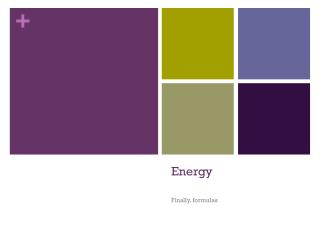Download PresentationEnergyEnergy - PowerPoint PPT Presentation

Download PresentationEnergy
An Image/Link below is provided (as is) to download presentation

Download Policy: Content on the Website is provided to you AS IS for your information and personal use and may not be sold / licensed / shared on other websites without getting consent from its author. While downloading, if for some reason you are not able to download a presentation, the publisher may have deleted the file from their server.

- - - - - - - - - - - - - - - - - - - - - - - - - - - E N D - - - - - - - - - - - - - - - - - - - - - - - - - - -
Presentation Transcript

1. Energy Finally, formulas

2. Energy • So we can’t exactly define energy, but we know we can measure it in some different forms • Kinetic • Gravitational • Elastic (springs) • Thermal (heat) • Electrical • Magnetic • Chemical

3. Measuring Energy • Energy is measured in the units of Joules (J) • 1J = 1 kg * m2 / s2 • So • J/kg = m2/s2 • J/(m2/s2)= Kg • N*m = J

4. Gravitational Potential Energy • The energy of height • On Earth: • GPE or Eg = m * g * h • m = mass in kg • g = 10m/s/s (gravitational constant) • h = height in m

5. How Much Energy does a 5kg object 2m off the ground have? • GPE = m * g * h • GPE = 5kg * 10m/s/s * 2m • GPE = 100 kg * m2/s2 • GPE = 100 J

6. Kinetic Energy • The energy of movement • KE or Ek = ½ m * v2 • m = mass in kg • v = velocity in m/s

7. How much kinetic energy does a 10kg car moving 4m/s have? • KE = ½ * m * v2 • KE = ½ * 10kg * (4m/s)2 • KE = 5kg * 16 m2/s2 • = 80 kg * m2/s2 • = 80J

8. Elastic Potential Energy (Eel) • Aka Spring Potential Energy (SPE) • Eel = ½ k * x2 • k = spring constant (how hard the spring is to compress) in N/m • x = how much the spring is stretched/compressed in m

9. How much Eel does a spring (constant = 100N/m) stretched 2m have? • Eel = ½ * k * x2 • Eel = ½ * 100N/m * (2m)2 • Eel = 50N/m * 4m2 • Eel = 200N*m • = 200J

10. Energy • So we can’t directly define or measure energy • But we can calculate the total amount of energy by adding all of the different forms • Total Energy = Ek + Eg + Eel + (other forms of energy)

11. Show your work! • On the classwork and homework and for ever and ever in physics you need to show your work • Allows you to do harder problems than the ones you have been given